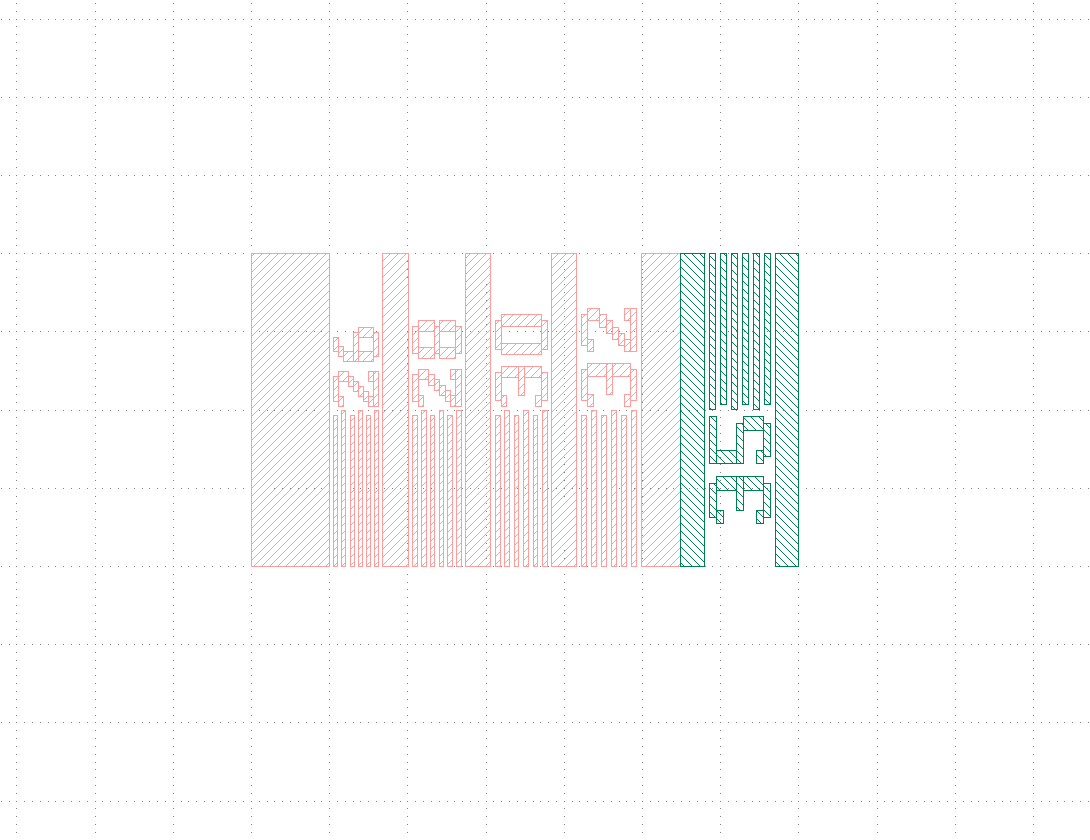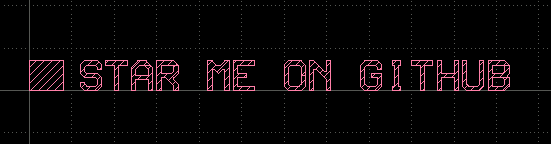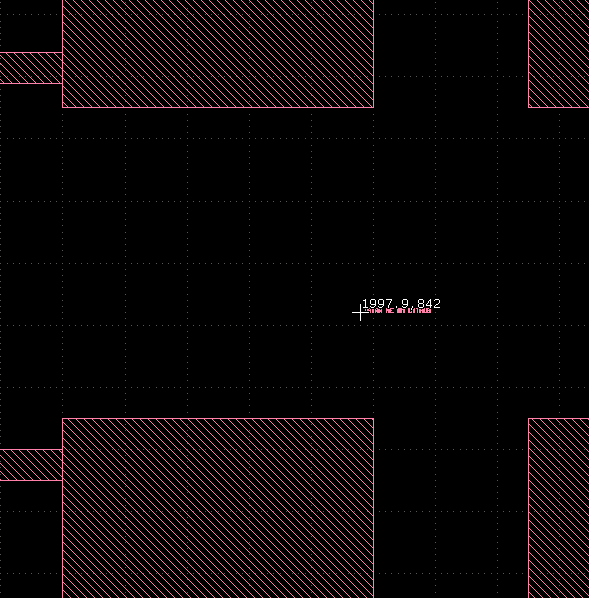# How to find a specific pattern

For resolution test of production process, a special pattern is inserted. The pattern is pre-defined but the location can vary. The pattern is composed of a few lines (rectagles with various but pre-defined dimensions) and a few characters drawn with rectangles.

How can a ruby script find the location of the pattern?

• Hi,

the solution strongly depends on how the pattern is defined. Could you how a sample?

Matthias

• Below is the pattern. Line widths are 26, 28, ..., 35um.• I have a proposal:

``````# reads pattern
pattern = pya.Layout()
# assumes the shapes are on layer 1/0
l1_pattern = pattern.layer(1, 0)
# gets the original pattern + merge to normalize areas
r_pattern = pya.Region(pattern.top_cell().begin_shapes_rec(l1_pattern)).merged()

layout = pya.Layout()

# find biggest rectangle of input pattern
# (this is assumed to be a good search seed)
area = 0
seed = None
for s in r_pattern.each():
if s.is_box() and s.area() > area:
area = s.area()
seed = s.bbox()
if not seed:
raise("No seed shape found")

# computes the pattern's bounding box as the pattern's definition area
# (assumes the pattern is rectangular)
pattern_box = r_pattern.bbox()

# look for seed shapes in the mask layout
# assumes the mask shapes are on layer 1/0
l1_layout = layout.layer(1, 0)
# select rectangles with the dimensions of the seed
dss = pya.DeepShapeStore()  # hierarchical mode

# now we got the seeds we can check where there is really the pattern we look for
for s in seeds.each():
offset = s.bbox().p1 - seed.p1
print("Checking position with offset " + str(offset) + " (DBU) ...")
around_seed = pya.Region(layout.top_cell().begin_shapes_rec_overlapping(l1_layout, pattern_box.moved(offset)))
# performs a clip to the pattern bounding box
clip = around_seed.moved(-offset) & pya.Region(pattern_box)
# does an XOR to confirm the pattern here
xor = clip ^ r_pattern
if xor.is_empty():
# we found the pattern
print("  -> CONFIRMED pattern here.")
else:
print("  -> REJECTED pattern here.")
``````

The idea is take the biggest rectangle from the pattern, look for the same on the mask and with these candidates do a detailed analysis using an XOR.

This was my test pattern:And this is where the script found it:Matthias